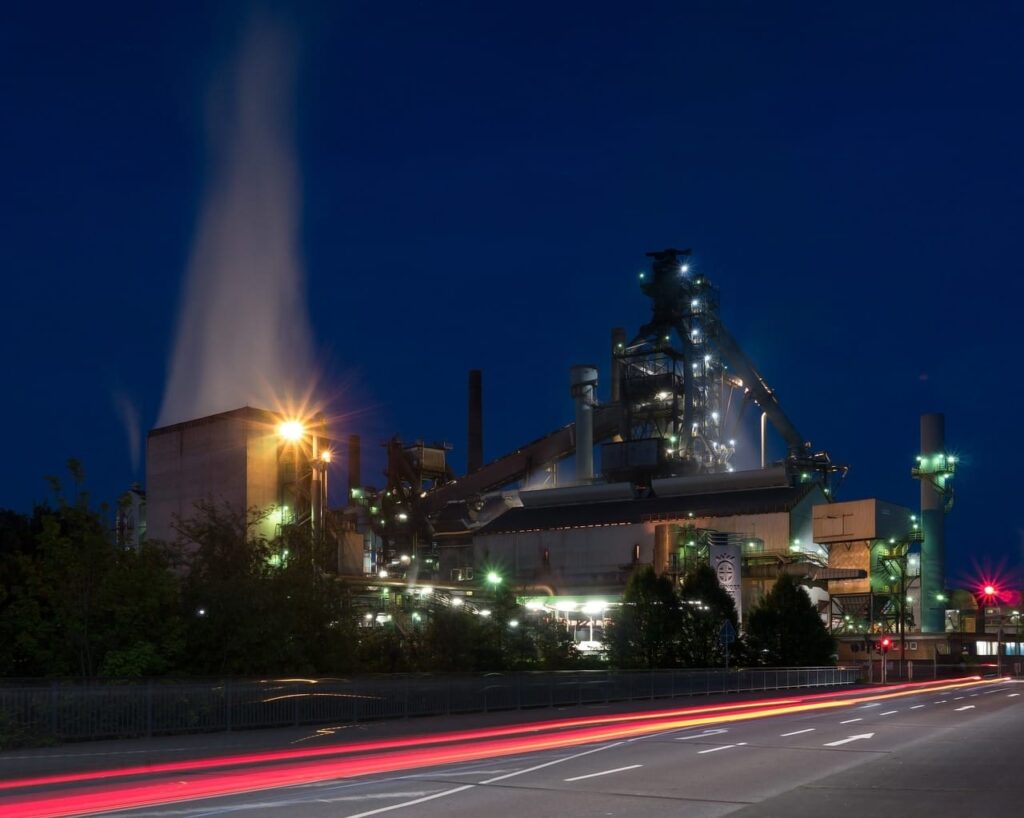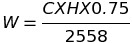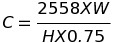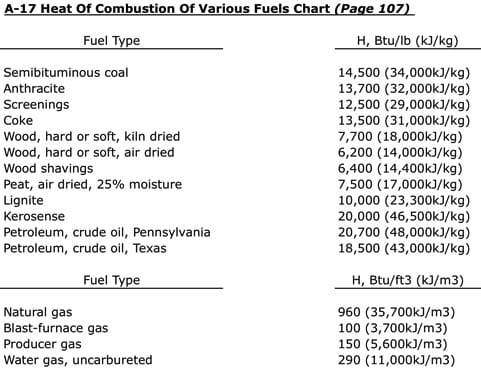# How To: Measure Pressure Relief Valve Capacity Dependent On Fuel Burned

Knowing How To: Measure Pressure Relief Valve Capacity Dependent On Fuel Burned will be useful throughout your Power Engineering career when completing boiler upgrades or conversions switching to a different fuel source. This will enable you to provide recommendations for the maximum amount of fuel able to be burned if the safety valves remain at their pre conversion settings or rerated.

All referenced page numbers are from the 2007 ASME Boiler & Pressure Vessel Code

## We Will Cover The Following

• How to measure pressure relief valve capacity dependent on fuel burned.
• Review the formula(s) provided in the Boiler & Pressure Vessel Code, along with formula(s) not provided.
• Explain the different variables used in each formula and which pages to find them on.
• Provide practice questions for you to review.

## Formula

Nonmandatory Appendix A A-12 page 103 The maximum quantity of fuel “C” that can be burned per hour at the time of maximum forcing is determined by a test. The maximum number of heat units per hour, or CH, is then determined, using the values of H given in A -17. The weight of steam generated per hour is found by the following equation:

Weight Of Steam Generated Per Hour EquationTotal Weight Or Volume Of Fuel Burned Per Hour EquationThe above formula to calculate the total weight or volume of fuel burned per hour at the time of maximum forcing is not provided in the 2007 ASME Boiler & Pressure Vessel Code

## Formula Variables

The variables used in the formulas provided above to determine how to measure pressure relief valve capacity dependent on fuel burned are defined in Nonmandatory Appendix A A-12 page 103

C = The total weight or volume of the fuel burned per hour at time of maximum forcing, lb/hr (kg/hr) or ft3/hr (m3/hr)

H = Heat of combustion of fuel, Btu/lb (kJ/kg) or Btu/ft3 (kJ/m3) (see A-17) page 107W = The weight of steam generated/hr, lb (kg/hr)

[table id=1 /]

## How To: Measure Pressure Relief Valve Capacity Dependent On Fuel Burned Questions

Note: All code questions are to be calculated in (mm) and (MPa) unless otherwise stated. Convert accordingly and properly before the calculation.

## Summary

Did the examples provided helped you understand How To: Measure Pressure Relief Valve Capacity Dependent On Fuel Burned?

Have you joined the Power Engineering 101 mailing list?

Thank you,

Power Engineering 101